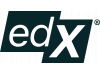date_range Inicia em 30 de janeiro de 2022
event_note Termina em 9 de junho de 2022
list 18 sequencias
chat_bubble_outline Idioma : InglêsEles escolhem Edflex para desenvolver suas habilidades na empresa.

Saiba mais
Avaliações
-
starstarstarstarstar
Ver avaliação

Informações principais

credit_card Free accesso
timer 126 total de horas

Sobre o conteúdo

Physicists use math all of the time in nearly everything that they work on. Hence, it is critical that you become efficient in being able to use more advanced math to enable you to work on more advanced physics courses. Most of you are currently what I would call a technician at math. You are able to perform the required manipulations to find and simplify answers. But, in many cases, you are missing the deeper understanding to help you interconnect different math topics and for you to be able to apply the relevant math for whatever problem you will face, based on your knowledge of how the different math topics inter-relate. On the other hand, a practitioner is someone who not only is technically adept at performing math calculations, but also have the insight and deeper understanding to know how to recognize what math applies to what problem. They understand how math is interconnected and recognize that math involves a handful of simple ideas that repeat. They are able to re-derive important formulas from basic principles or know precisely where to look them up and use them.

The goal of this class is to transform you from a math technician to a math practitioner. Mathematicians take this one step further and actually create new math. We will not focus on how to do that at all in this class.

more_horiz Ler mais
more_horiz Ler menos
dns

Programa de estudos

• Be able to apply techniques of calculus (learned in the first three semesters of a calculus sequence) to solve problems that arise in physics.
• Derive and use the geometric series in calculations.
• Manipulate power series expressions and employ them in physics contexts.
• Calculate Taylor polynomials/series of common functions and use them in approximating functions
• Follow the development for how one integrates polynomials, rational functions of polynomials, square roots of quadratics, rational functions with square roots of quadratics, and why the procedure cannot solve integrals with square roots of quartics.
• Solve integrals via parametric methods (differentiating under the integral sign) including techniques for introducing the parameter into the integrand
• Set up and integrate multidimensional integrals with variable mass density and for moments of inertia.
• Solve problems in multivariable integrals via the different integral theorems
• Solve Laplace's equation for simple geometries
• Compute the curl or divergence of a vector field and the gradient of a scalar function
• Solve complex numbers arithmetic problems
• Use calculus of residues to solve integrals
• Use row reduction to solve simultaneous linear equations
• Calculate the determinant, inverse, and eigenvalues of matrices
• Construct abstract vector spaces using the definitions of a vector space and generalized inner products
• Solve any first-order linear differential equation
• Solve the six classes of nonlinear first-order differential equations
• Solve higher-order differential equations with constant coefficients (homogeneous and inhomogeneous)
• Construct representations of curves in three dimensions using the Frenet-Serret apparatus
• Construct Fourier series of periodic functions
record_voice_over

Instrutores

James Freericks
Professor
Georgetown University

assistant

PlataformaEdX est une plateforme d'apprentissage en ligne (dite FLOT ou MOOC). Elle héberge et met gratuitement à disposition des cours en ligne de niveau universitaire à travers le monde entier. Elle mène également des recherches sur l'apprentissage en ligne et la façon dont les utilisateurs utilisent celle-ci. Elle est à but non lucratif et la plateforme utilise un logiciel open source.

EdX a été fondée par le Massachusetts Institute of Technology et par l'université Harvard en mai 2012. En 2014, environ 50 écoles, associations et organisations internationales offrent ou projettent d'offrir des cours sur EdX. En juillet 2014, elle avait plus de 2,5 millions d'utilisateurs suivant plus de 200 cours en ligne.

Les deux universités américaines qui financent la plateforme ont investi 60 millions USD dans son développement. La plateforme France Université Numérique utilise la technologie openedX, supportée par Google.

Você é o criador deste MOOC?
Qual a sua apinião sobre esse recurso?
Conteúdo
5/5
Platforma
5/5
Didática
5/5